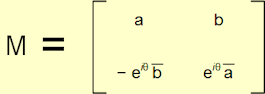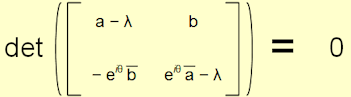# Dropped Text

 First recall that in Chapter 14 we demonstrated (with some effort it must be said) that the generic form of a 2 × 2 Unitary matrix, M, is:Where a and b ∈ ℂ, θ is an angle with 0 ≤ θ < 2π and |a|2 + |b|2 = 1. We can use this to form our modified matrix, M – λI as follows:We then recall the requirement that the determinant of this matrix is zero:Again the determinant of a 2 × 2 matrix with entries {a, b, c, d} is simply ad – bc, so we have: (a – λ)(eiθ a – λ) + b eiθ b = 0 Multiplying out, we get: a eiθ a – aλ – λ eiθ a + λ2 + b eiθ b = 0 Regrouping: λ2 – λ(a + eiθ a) + (a eiθ a + b eiθ b) = 0 Or: λ2 – λ(a + a eiθ) + (a a eiθ + b b eiθ) = 0          (1) From the definition of Unitary matrices, we know that |a|2 + |b|2 = 1 and from Chapter 14 we can recall that for any complex number, z, we have: z z = |z|2 This means that: a a + b b = 1 Or: b b = 1 – a a Substituting this in (1) above, we get: λ2 – λ(a + a eiθ) + (a a eiθ + (1 – a a) eiθ) = 0 Multiplying out the bracket again we get: λ2 – λ(a + a eiθ) + (a a eiθ + eiθ – a a eiθ) = 0 Cancelling the ± a a eiθ, we get: λ2 – λ(a + a eiθ) + eiθ = 0          (2) Once more in Chapter 14 we also established that for a complex number, z, which forms an angle φ with the x-axis, we have: z = |z| eiφ and z = |z| e– iφ If we substitute both a and a with this formulation in (2), we get: λ2 – λ(|a| eiφ + |a| e– iφ eiθ) + eiθ = 0 Which we can simplify to: λ2 – λ|a|(eiφ + ei(θ – φ)) + eiθ = 0

FROM CHAPTER 21

Back in Chapter 17, we showed that the Cayley-Hamilton Theorem for a 3 × 3 matrix, M3, implies that:

M33 – tr(M3)M32 + ½[tr(M3)2 – tr(M32)]M3 – det(M3)I3 = 0

Where I3 is the 3 × 3 identity matrix.

If we consider a matrix S ∈ su(3), then by definition its trace is zero, so the terms involving tr(S) in the above drop out and we get:

S3 – ½tr(S2)S – det(S)I3 = 0

or

S3 = ½tr(S2)S + det(S)I3          (1)

Back in Chapter 18, we noted that:

tr(M + N) = tr(M) + tr(N)          (2)

and

tr(αM) = α tr(M)          (3)

Where α is a scalar.

If we take the trace of both sides of (1), we get:

tr(S3) = tr[½tr(S2)S + det(S)I3]

Using (2) we note that:

tr(S3) = tr[½tr(S2)S] + tr[det(S)I3]

Using (3) and noting that the trace of a matrix is a scalar we have that:

tr(S3) = ½tr(S2)tr(S) + det(S)tr(I3)

Again tr(S) is zero and obviously tr(I3) = 3, so:

tr(S3) = 3det(S)

or

det(S) = tr(S3)/3